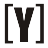# Lux to lumens conversion calculator

 Enter Illuminance in lux: lx Select area unit type: Area in square feet Area in square meters Enter spherical radius: Or enter surface area: Luminous flux result in lumens: lm

## Lux to lumens manual calculation

area in square feet
luminous flux ΦV in lumens (lm) is equal to 0.09290304 multiplied by illuminance Ev in lux (lx) multiplied by the surface area A in square feet (ft2)

`ΦV(lm) = 0.09290304 × Ev(lx) × A(ft2)`

luminous flux ΦV in lumens (lm) is equal to 0.09290304 times the illuminance Ev in lux (lx) times 4 times pi times the squared sphere radius r in feet (ft):

`ΦV(lm) = 0.09290304 × Ev(lx) × 4⋅π⋅r(ft) 2`

area in square meters

luminous flux ΦV in lumens (lm) is equal to the illuminance Ev in lux (lx) multiplied by surface area A in square meters (m2)

`ΦV(lm) = Ev(lx) × A(m2)`

luminous flux ΦV in lumens (lm) is equal to the illuminance Ev in lux (lx) multiplied by 4 times pi times the squared sphere radius r in meters (m)

`ΦV(lm) = Ev(lx) × 4⋅π⋅r 2(m2)`YoosFuhl.com Would you like to receive notifications on latest updates? No Yes